Science, Maths & Technology

### Become an OU student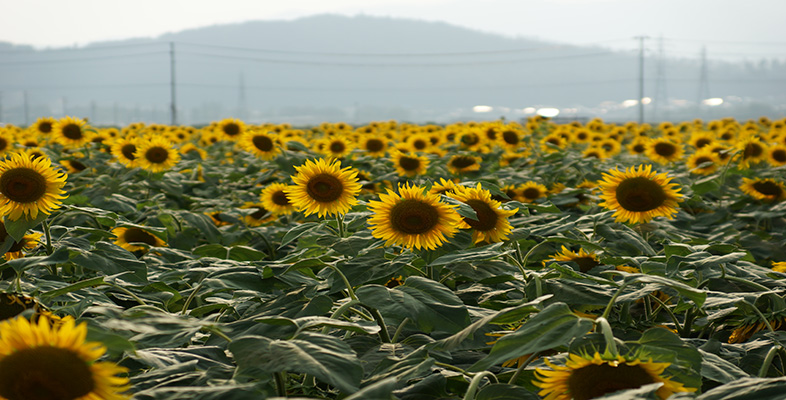Ratio, proportion and percentages

Start this free course now. Just create an account and sign in. Enrol and complete the course for a free statement of participation or digital badge if available.

# 3.3 Percentage increase and decrease

## 3.3.1 Increasing by a percentage

Our everyday experience of percentages includes percentage increases (like VAT at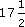%, or a service charge of 15%) and percentage decreases (such as a discount of 15%).

For example, £8 plus% VAT means you actually have to pay

£8 + (% of £8).% of 8 is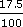× 8 =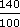= 1.4

so the VAT is £1.40, and the sum you pay is £8 + £1.40 = £9.40.

There is another way of doing this calculation:

100% of £8 plus% of £8 = (100% +%) of £8,

that is 117.5% of £8.

117.5% of £8 = £(1.175 × 8)

= £9.40 (as before).

### Example 12

A restaurant bill comes to £76 before VAT and the service charge are added. VAT is added atand the restaurant also adds a service charge of 15%. Does it make any difference to what you have to pay if the VAT is added first then the service charge or vice versa? Does it make a difference to the amount of VAT paid?

Adding VAT first gives 100% +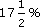=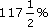of £76 = 1.175 × £76 = £89.30

Adding the service charge on to £89.30 gives 100% + 15% = 115%

115% of £89.30 = 1.15 × £89.30 = £102.70 (correct to the nearest penny).

So the total bill is £102.70.

Adding these extras the other way round:

115% of £76 = 1.15 × £76 = £87.40;of £87.40 = 1.175 × £87.40

= £102.70 (correct to the nearest penny).

In the first case 1.15 × 1.175 × £76, and in the second 1.175 × 1.15 × £76. The order doesn’t matter in multiplication: 1.175 × 1.15 = 1.15 × 1.175.

The total bill is the same. The order does not matter from the customer's point of view. However, it does from the VAT collector's point of view (and indeed from that of the restaurant management, who receive a bigger service charge if it is calculated last). If VAT is added first, VAT isof £76. If VAT is added last, VAT isof £87.40. Hence legally VAT must be added last!